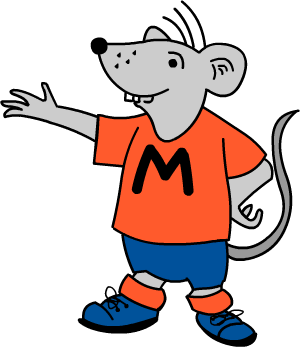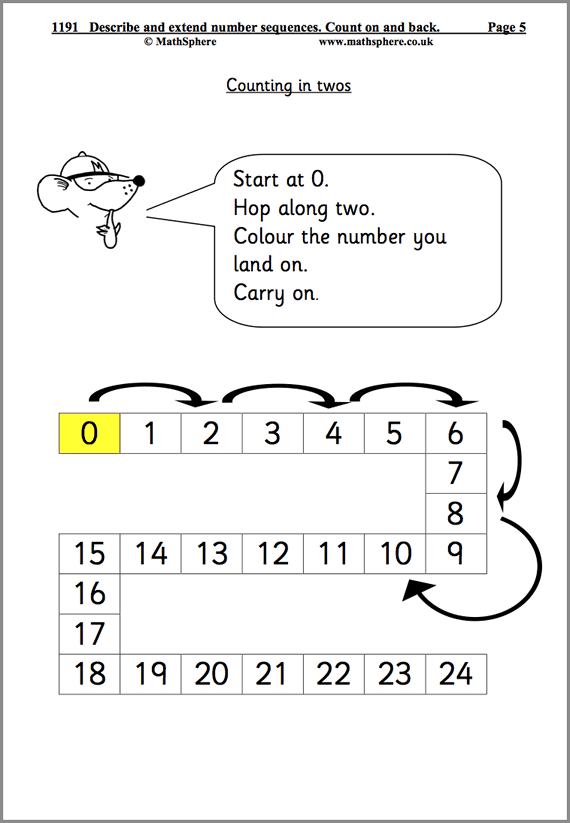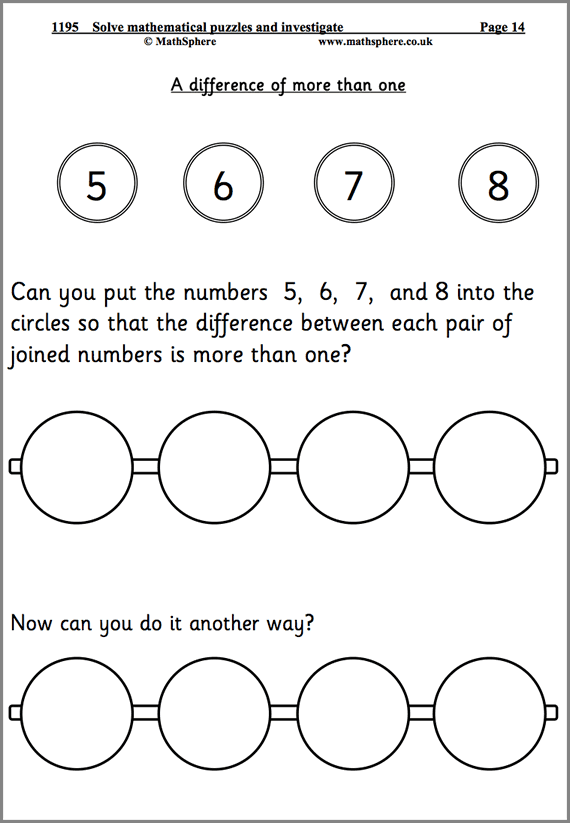# Year 1 Maths Worksheets

A superb set of worksheets covering all aspects of Mathematics in Year 1 (aged 5‐6). Each page has been individually written to give children a great start to their mathematics education. An invaluable resource for both teachers and parents.

## The Very Best Year 1 Maths Worksheets

Starting with simple counting in steps of one and moving right through to more complex maths such as recognising fractions and solving problems involving money, there are over 700 pages in PDF format to choose from.

• Updated for the very latest statutory requirements with many new pages added
• Answers included where necessary to make marking simple
• Single user licence for teachers or parents (school site licence upgrade available)
• Concept pages clearly explain the ideas being taught
• Written by experienced primary teachers
• MathSphere worksheets are already being used in over 10 000 primary schools## Try Some Free Sample Worksheets

To give you a better idea of the worksheets available we have provided a selection of free modules for Year 1.

Please download these samples, print them out and use at home, or photocopy and use in the classroom.24 Pages

#### Number Sequences

Using number lines to hop along in twos, threes and fives as well as extending number sequences.14 Pages

Adding and subtracting ten from numbers up to 20.19 Pages

#### Solve Problems

Children use their knowledge to solve mathematical problems and puzzles – great fun!

## List of modules

It is impossible to describe in a single page the massive amount of high quality maths contained in this one product.

To give you some idea, have a look at the list of all modules:

### Number and place value 265 pages

 Count reliably a set of objects. Count in steps of one. 16 Read and write numbers in figures and words. 22 Counting over ten. 22 Comparing and ordering numbers. More than, less than. 20 Counting in twos. 23 One or ten more than, or less than a given number. 16 Counting in tens; odd and even numbers. 14 Counting in fives. 6 Count back in ones, twos and tens. 15 Order a set of familiar numbers. 16 Describe and extend number sequences. 24 Estimation and approximation. 13 Know the number names and recite them. 17 Nursery rhymes with number and counting. 22 Solve mathematical puzzles and investigate. 19

### Multiplication and division 56 pages

 Doubles and halves. 22 Combining groups of 2, 5 and 10. 16 Using arrays. 7 Understanding division; sharing into equal groups. 11

### Measures 91 pages

 Measure and compare. 9 Suggest suitable units to estimate and measure. 13 Use simple measuring equpiment. 10 Understand and read the time. 18 Word problems involving measurement. 20 Problems involving money. 21

### Mental arithmetic 72 pages

 Autumn term. 24 Spring term. 24 Summer term. 24

### Addition and subtraction 181 pages

 Understand addition. 12 Understand subtraction. 11 Know addition and subtraction facts. 24 Add or subtract 9. 20 Add or subtract pairs of numbers mentally. 23 Understand that more than two numbers can be added together. 12 Use knowledge that addition can be done in any order. 8 Adding or subtracting 10. 14 Adding or subtracting crossing the tens. 15 Add or subtract a multiple of 10. 13 Choose and use appropriate number operations to solve problems. 8 Investigate general statements. 10 Solve simple word problems. 11

### Fractions 25 pages

 Recognise simple fractions and equivalence. 15 Recognise and find simple fractions. Count in halves. 10

### Geometry 40 pages

 Describe 3D and 2D shapes. 16 Make models and describe the features of shapes. 11 Describe position and direction. 7 Describe movement and turning. 6

### Using and Applying Mathematics 29 pages

 Length, number and ordering word searches. 8 Investigate 3 digits. 5 Investigate totals to 20. 5 Caterpillar investigation. 5 Penny sweets investigation. 6

### Statistics 23 pages

 Collect and sort information (1) 12 Collect and sort information (2) 11

### Quick Details

• Over 700 worksheets
• Concept pages included
• For ages 5 – 6
• PDF format